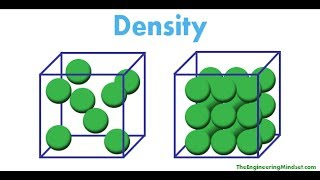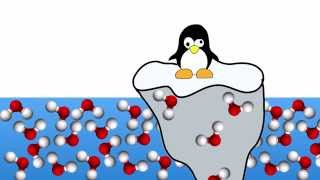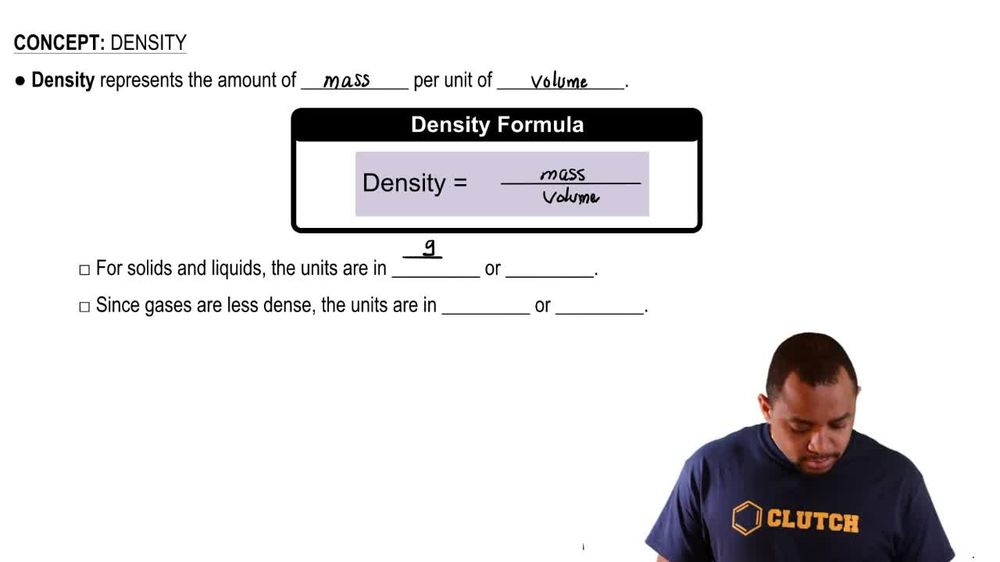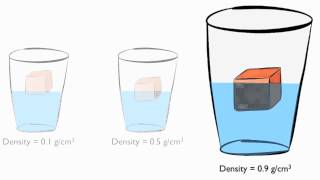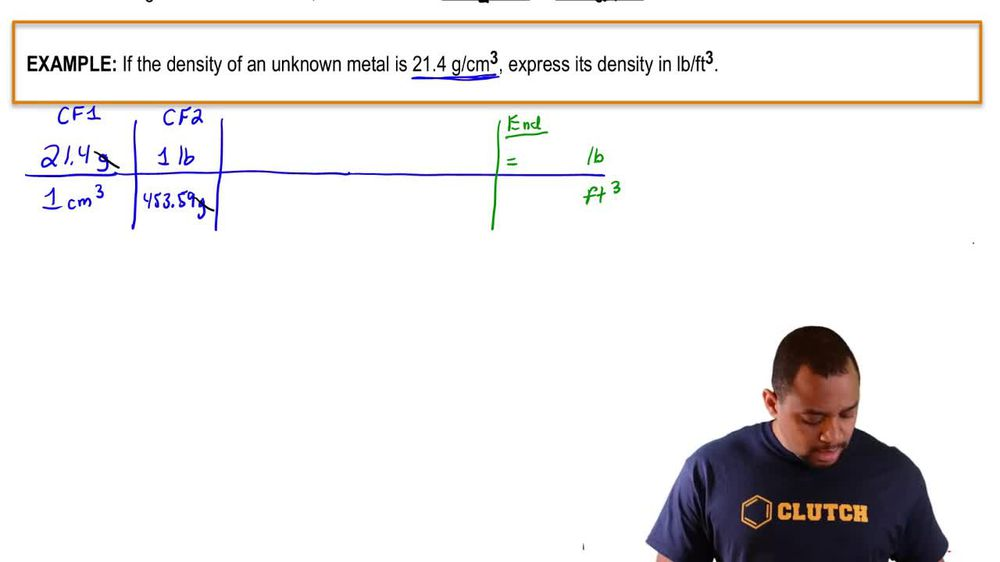Start typing, then use the up and down arrows to select an option from the list.
1. 1. Intro to General Chemistry2. Density
Problem

# What is the density of glass in g/cm3 if a sample weighing 27.43 g has a volume of 12.40 cm3?

Relevant Solution1m
Play a video:
Hi everyone today, we have a question telling us that an unknown 15 millimeter sample of liquid at room temperature was determined to weigh 69.3 g, calculate its density and determine if it will float on water. So for this we're going to use the equation mass equals volume times density. And we want to solve for density. So density will equal mass divided by volume. So now, plugging in what we know. Our density equals 69 0. g, divided by 15 mL, And that equals 4. grams per middle leader. And we're going to assume that water has a density Of about one g per mil leader. 4. Is greater than one, so it will sink. And there are our final answers. Thank you for watching. Bye.